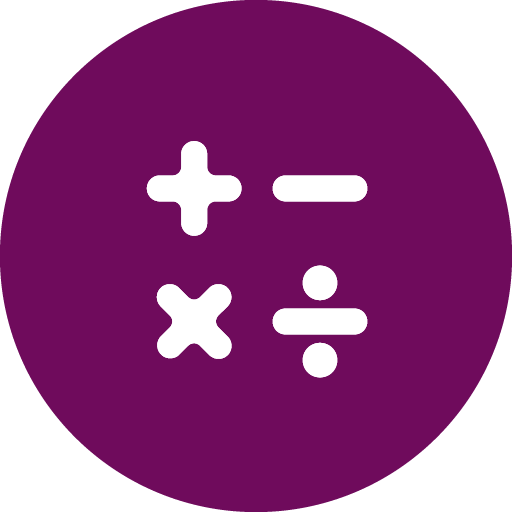#calculatorSensor v0.0.5

Simple arithmetic calculator based on the input values.

Input values is either a list of numbers or list of objects. If the input array is list of objects, you need to provide a key for which will this sensor perform mathematical operation.

Operator can be

• min
• max
• sum
• mean

For instance

```values: [ {“key1”: {“key2”:1}}, {“key1”: {“key2”:5}}]
key: key1.key2
operator: sum
```

# Required properties:

• `values`
• `key`
• `operator`

# States:

• `done`
• `error`

# Raw data:

• `result (double)`
• `operator (double)`